## Notched I-TetrominoesThe picture above shows a construction with square symmetry using all 32 I-tetrominoes with three notches.

For two-sided and one-sided I-tetrominoes with a given number of notches the number of different pieces and some possible constructions are listed in the following table.

Number of Notches Number of Pieces Total Area Constructions
Two-sided Pieces k odd 1/4*(10Ck + 2*(4C((k-1)/2))) 10Ck + 2*(4C((k-1)/2))
k even 1/4*(10Ck + 3*(5C(k/2))) 10Ck + 3*(5C(k/2))
1 3 12 No constructions, because the total number of notches is odd
2 15 60 No rectangles
3 32 144 8x16 rectangle, some symmetric figures
One-sided Pieces k odd 1/2*(10Ck) 2*(10Ck)
k even 1/2*(10Ck + (5C(k/2)) 2*(10Ck + (5C(k/2))
1 5 20 No constructions, because the total number of notches is odd
2 25 100 5x20 rectangle
3 60 240 8x30, 10x24, 12x20 and 15x16 rectangles, square ring

There are 15 two-sided pieces with two notches and the set has a total area of 60. You can't get a 6x10 rectangles, even if notches are ignored, because neither 6 nor 10 is a multiple of 4. The borders of the 5x12 and 4x20 rectangles seem to be too long and an exhaustive search showed, that these rectangles are impossible to cover, if straight borders and aligned notches are demanded.

Let's have a look at the 32 I-Tetrominoes with three notches. The picture shows a rectangles of size 8x16. The pieces are cut from black hard foam board with yellow adhesive triangles attached.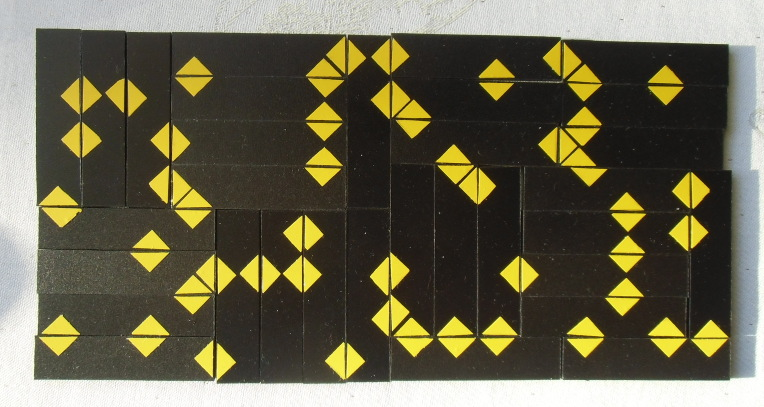Beside the figure with square symmetry in the title there are some other figures with one or two axis of symmetry or rotational symmetry.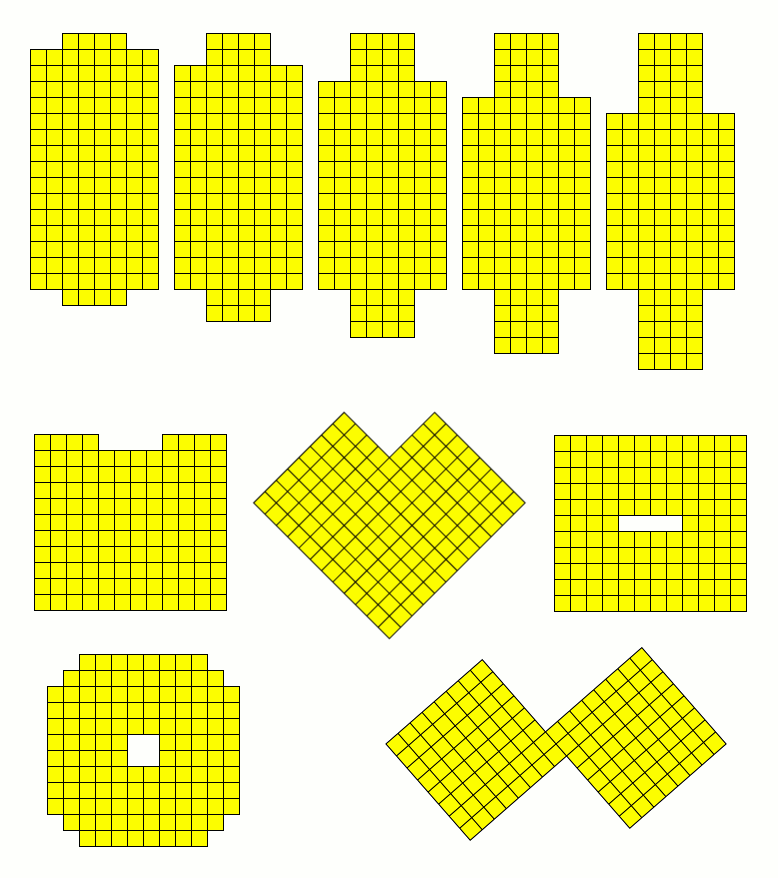The solutions are here.

The total area of the 25 one-sided I-tetrominoes with two notches ist 100. You may ask to pack a 10x10 square with these pieces, but this is impossible since the length of both sides is 10, which isn't a mutiple of 4. At least one side must be divisible by 4 as shown by Andy Liu . A 5x20 rectangle is filled with no problem.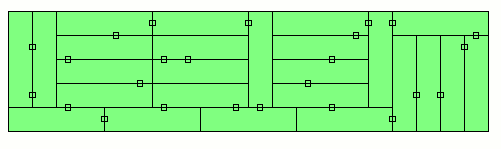There are 60 one-sided I-tetrominoes with three notches giving a total area of 240. This number factorizes very well and rectangles of size 8x30, 10x24, 12x20 and 15x16 can be covered.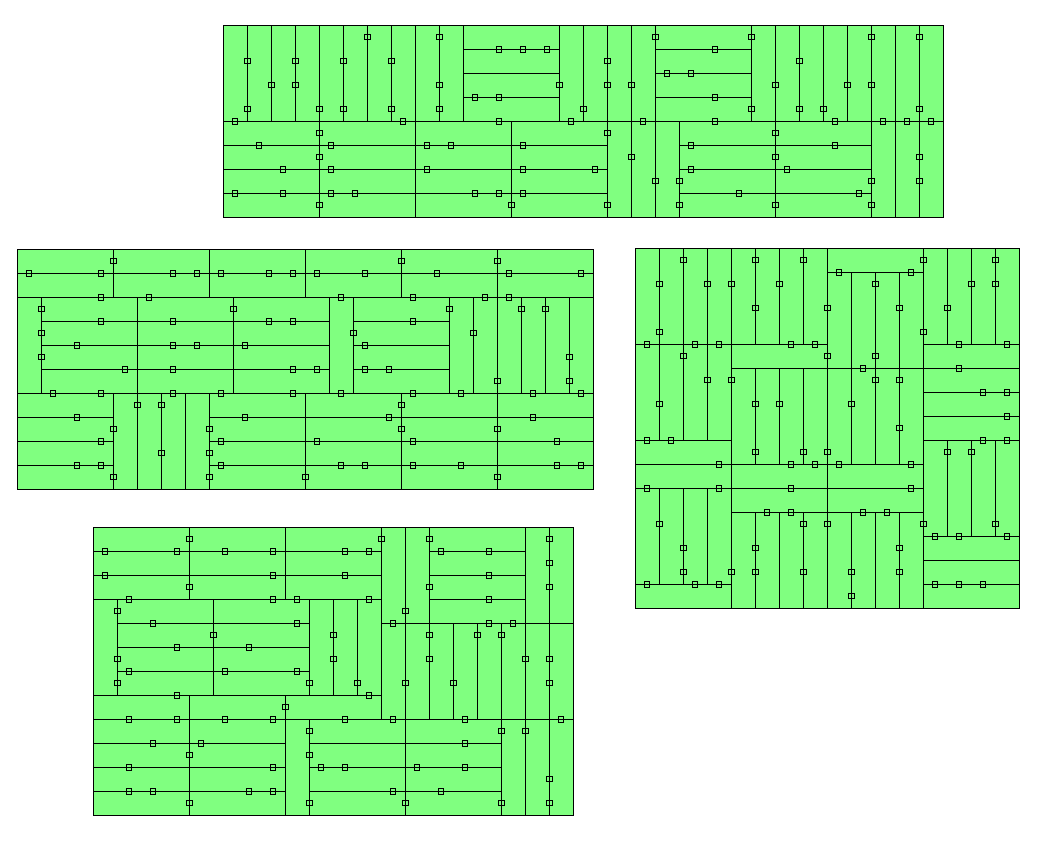A figure with square symmetry is a ring of size 16˛ - 4˛.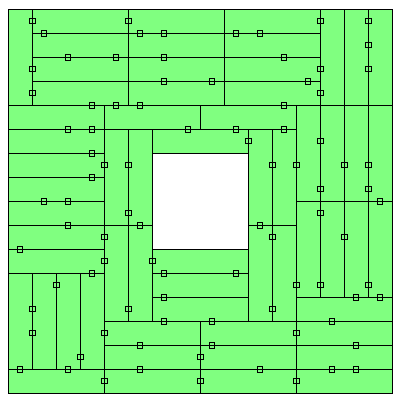Back

Home